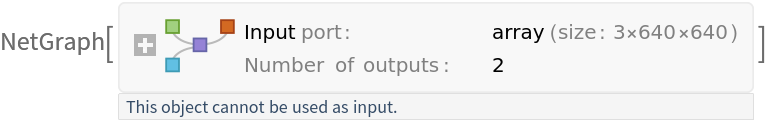# YOLO V8 Detect Trained onMS-COCO Data

Detect and localize objects in an image

YOLO (You Only Look Once) Version 8 by Ultralytics is the latest version of the YOLO models. Just like its predecessor, YOLO Version 5, YOLO Version 8 is an anchor-free model that was trained with mosaic augmentation. It features the use of new "C2f" blocks, which employ additional dense connections between bottleneck modules. YOLO Version 8 models outperform all models from previous versions at a similar size.

## Training Set Information

• Microsoft COCO, a dataset for image recognition, segmentation, captioning, object detection and keypoint estimation, consisting of more than three hundred thousand images.

## Model Information

•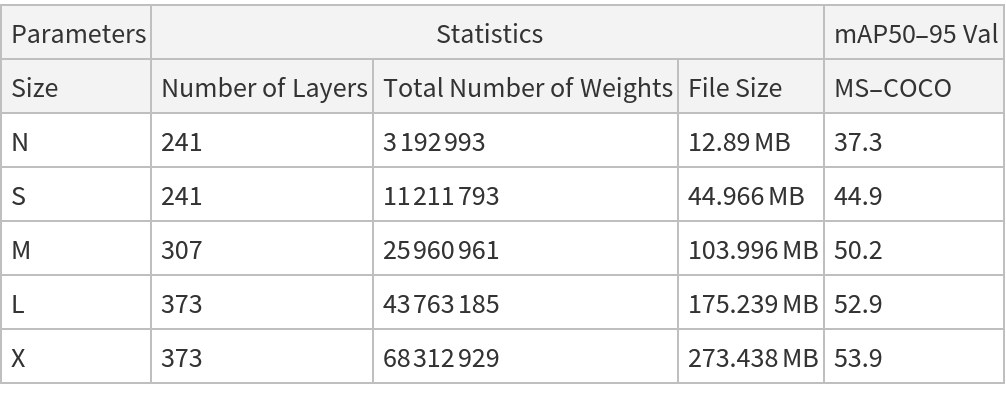## Examples

### Resource retrieval

Get the pre-trained net:

 In:=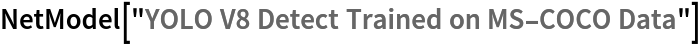Out=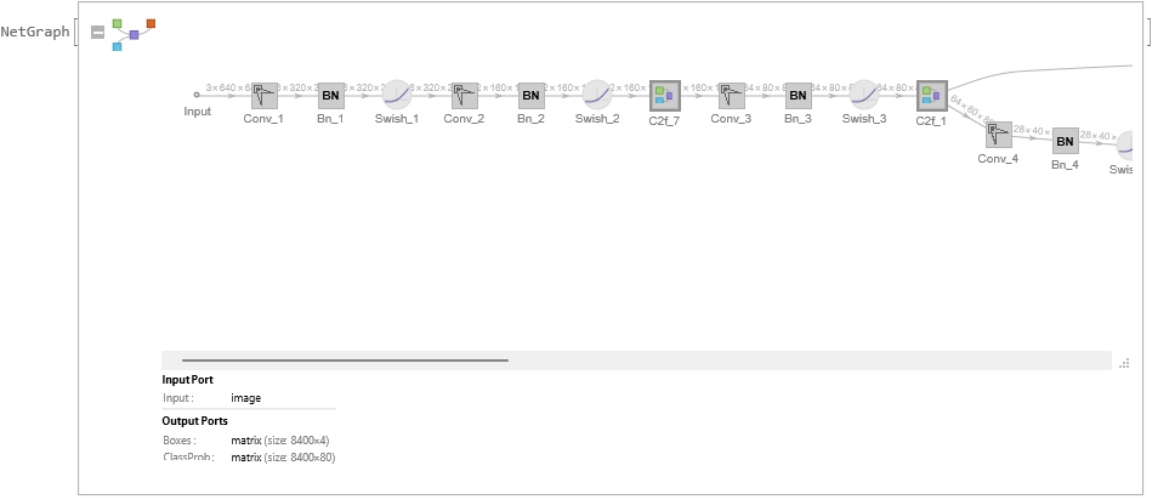### NetModel parameters

This model consists of a family of individual nets, each identified by a specific parameter combination. Inspect the available parameters:

 In:=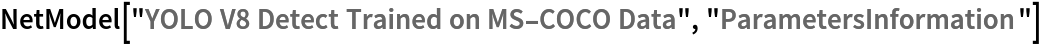Out=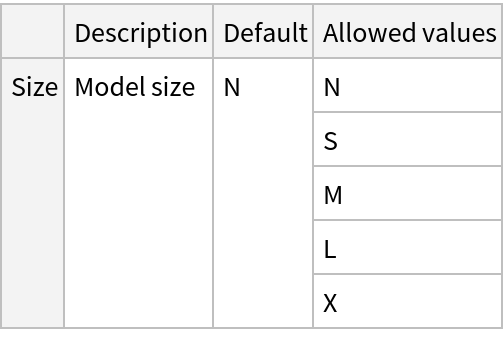Pick a non-default net by specifying the parameters:

 In:=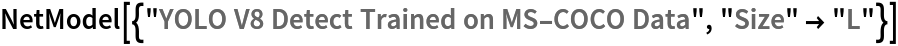Out=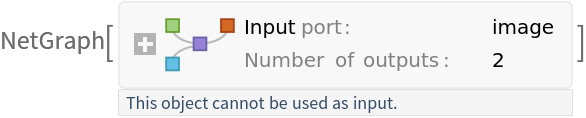Pick a non-default uninitialized net:

 In:=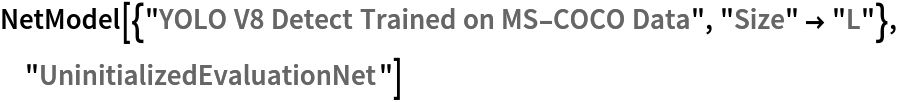Out=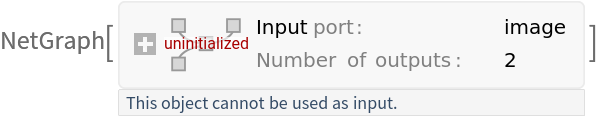### Evaluation function

Write an evaluation function to scale the result to the input image size and suppress the least probable detections:

 In:=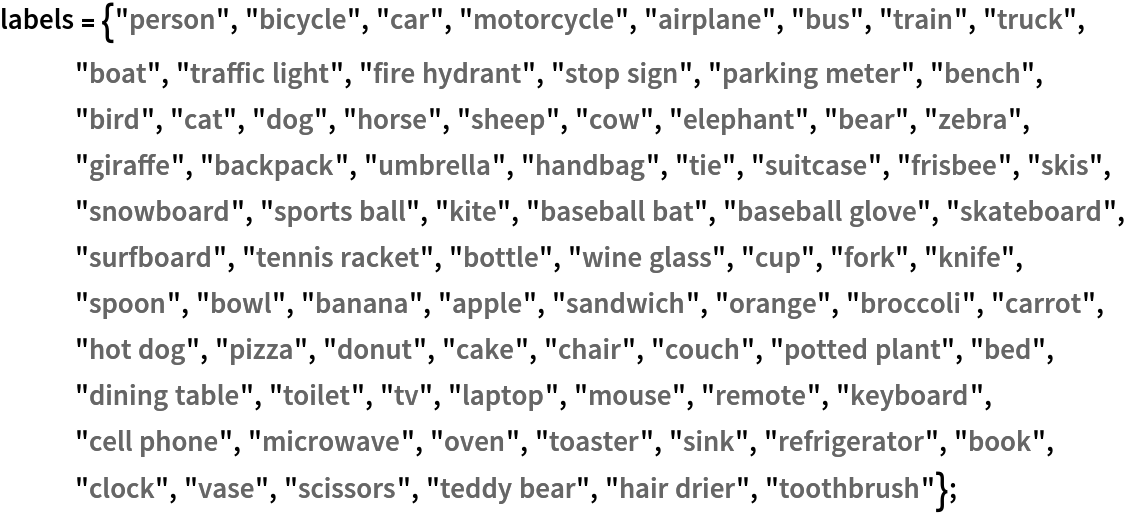In:=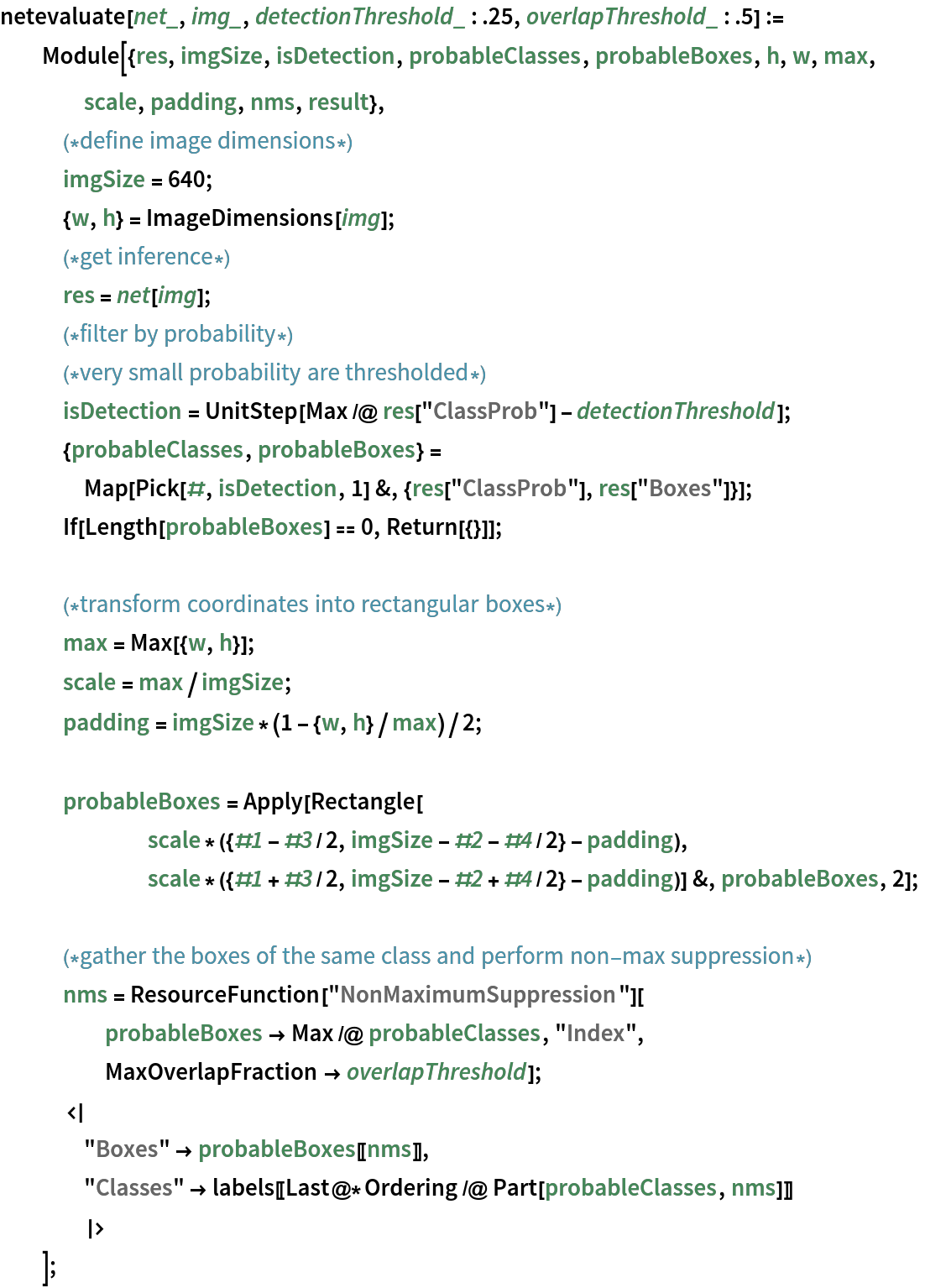### Basic usage

Obtain the detected bounding boxes with their corresponding classes and confidences for a given image:

 In:=In:=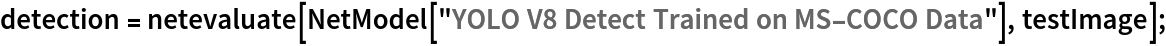The model's output is an Association containing the detected "Boxes" and "Classes":

 In:=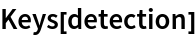Out=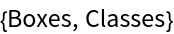The "Boxes" key is a list of Rectangle expressions corresponding to the bounding boxes of the detected objects:

 In:=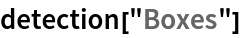Out=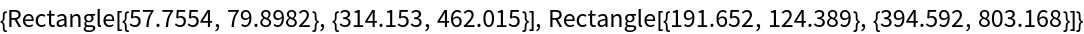The "Classes" key contains the classes of the detected objects:

 In:=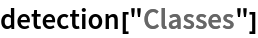Out=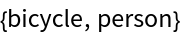Visualize the detection:

 In:=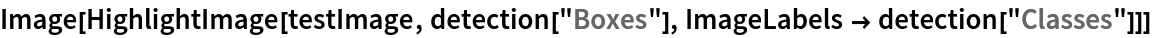Out=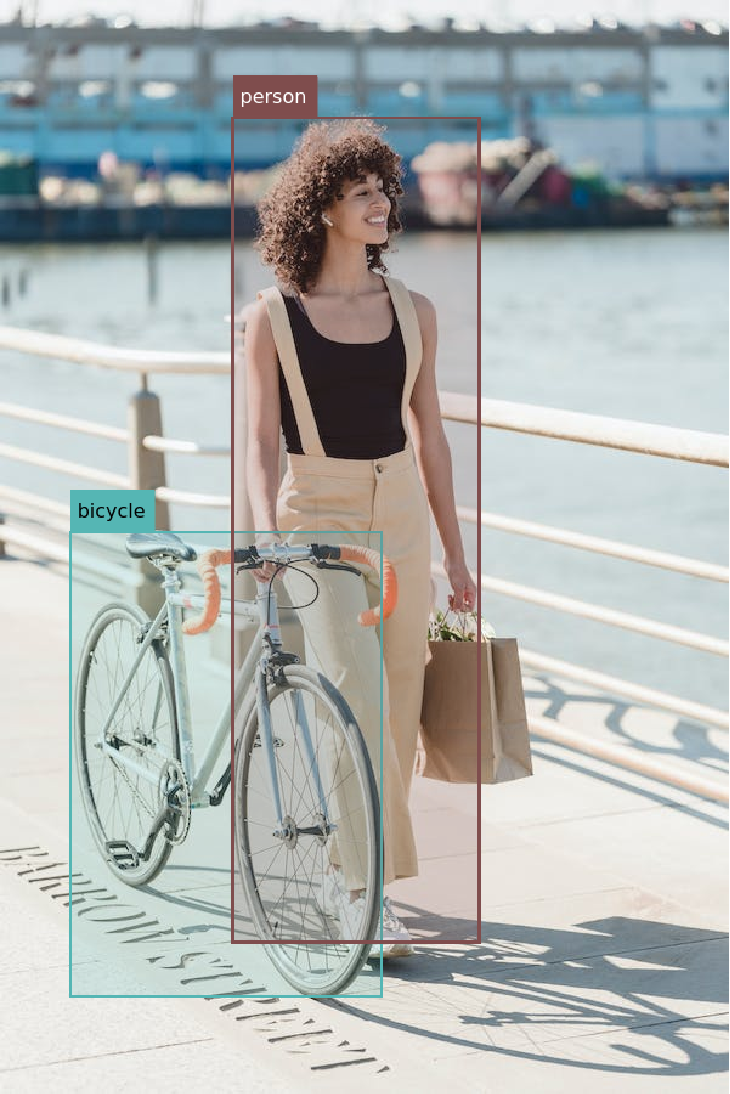### Network result

The network computes eight thousand four hundred bounding boxes and the probability that the object is of any given class:

 In:=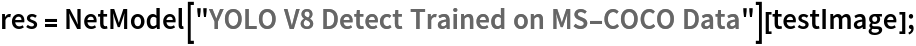In:=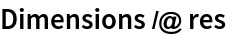Out=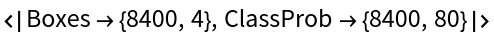Visualize the bounding boxes scaled by their class probabilities:

 In:=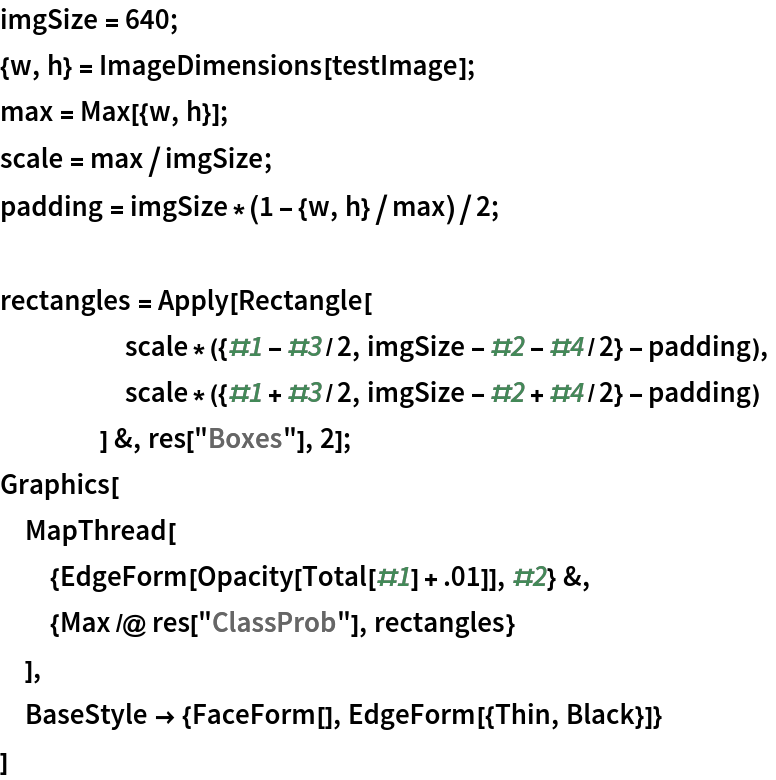Out=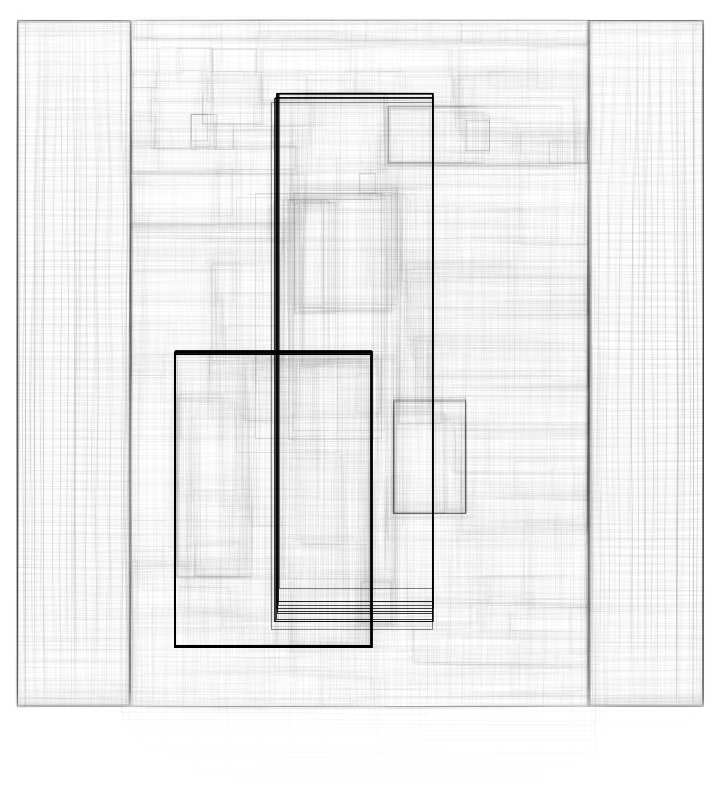Visualize all the boxes scaled by the probability that they contain a person:

 In:=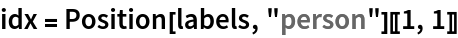Out=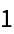In:=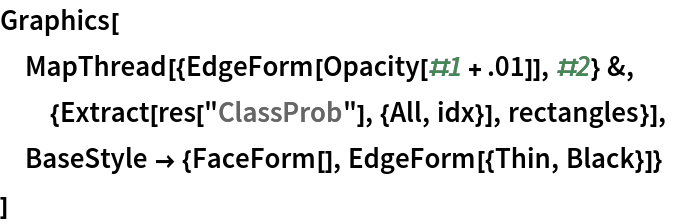Out=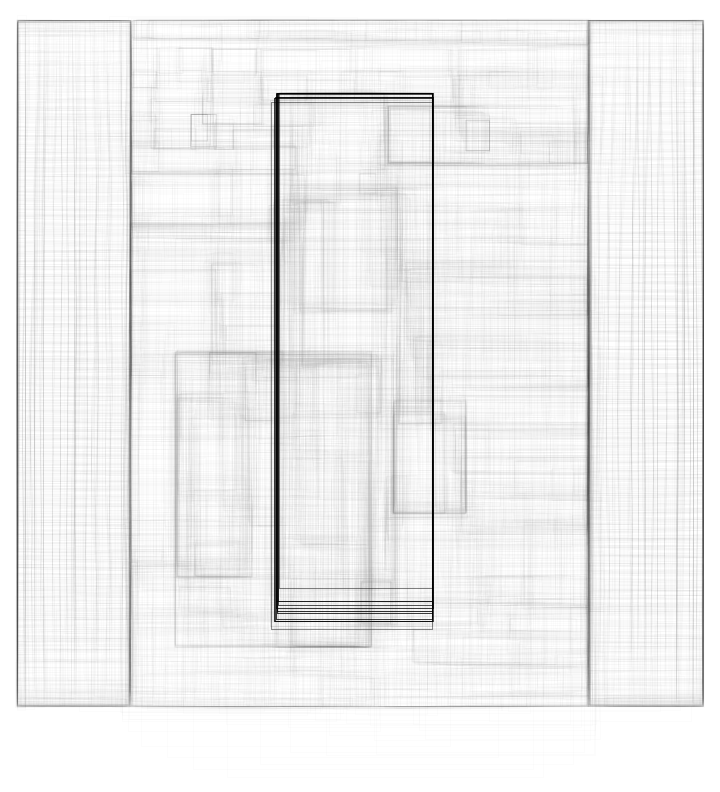Superimpose the person predictions on top of the input received by the net:

 In:=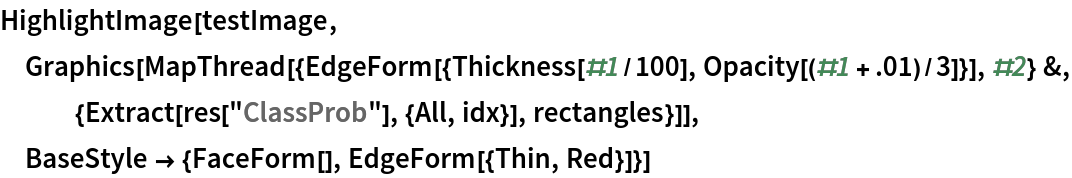Out=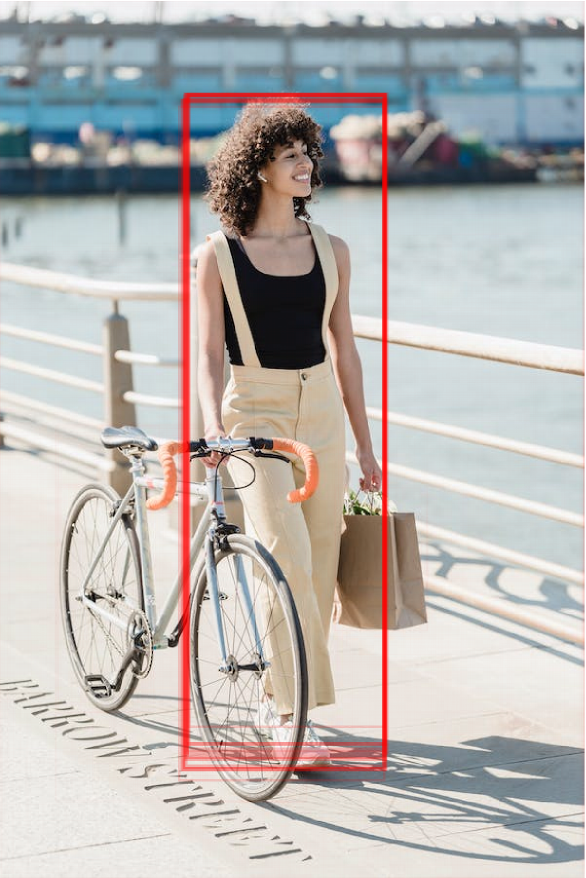### Net information

Inspect the number of parameters of all arrays in the net:

 In:=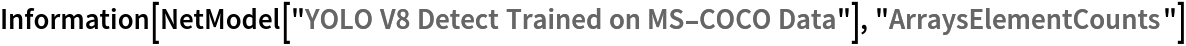Out=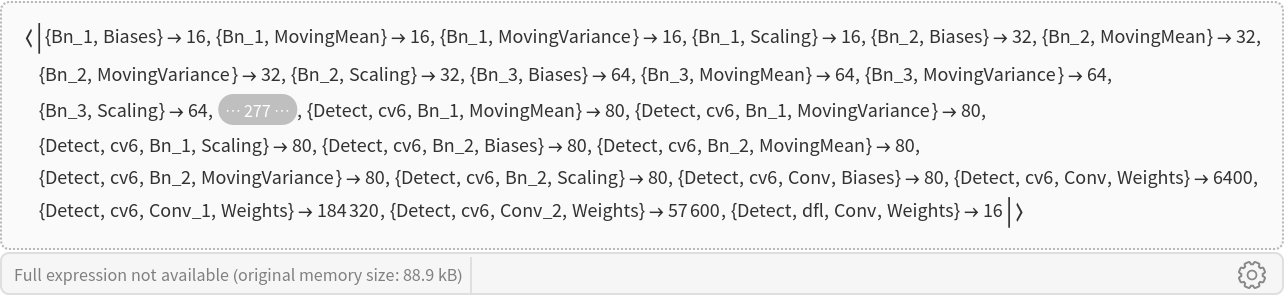Obtain the total number of parameters:

 In:=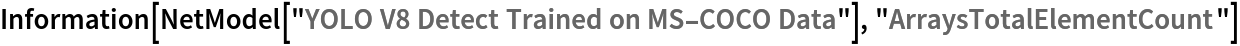Out=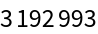Obtain the layer type counts:

 In:=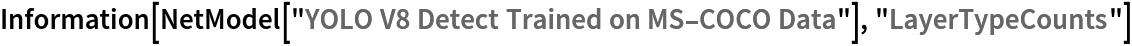Out=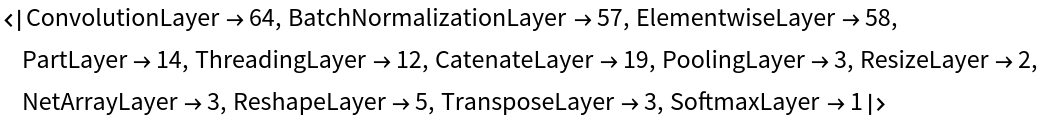Display the summary graphic:

 In:=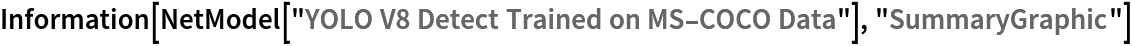Out=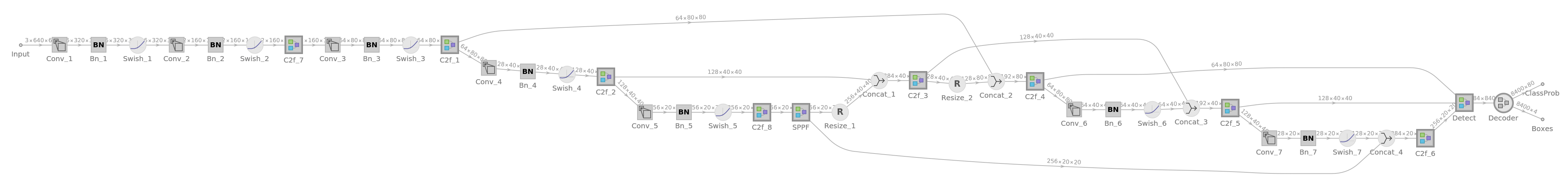### Export to ONNX

Export the net to the ONNX format:

 In:=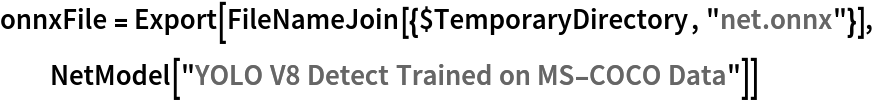Out=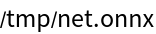Get the size of the ONNX file:

 In:=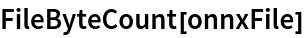Out=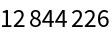The size is similar to the byte count of the resource object:

 In:=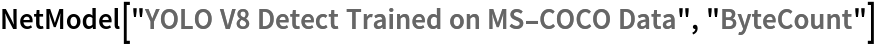Out=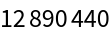Check some metadata of the ONNX model:

 In:=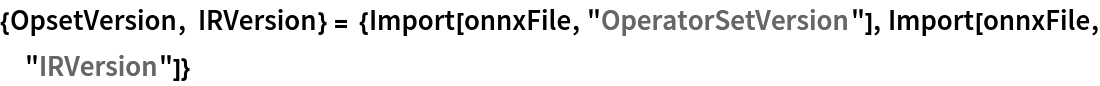Out=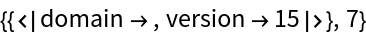Import the model back into Wolfram Language. However, the NetEncoder and NetDecoder will be absent because they are not supported by ONNX:

 In:=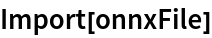Out=